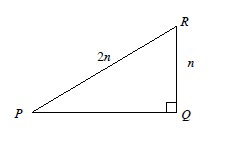### Home > PC > Chapter 3 > Lesson 3.3.2 > Problem3-128

3-128.

Using $\triangle PQR$ below, complete the following problems.Review problems 3-74 and 3-112.

1. Find the length of $\overline{P Q}$ in terms of $n$.

2. Find $\sin P$.

3. Find $\cos P$.

4. What is the measure of $\angle P$?

5. Since $n$ always cancels in parts (b) and (c), what can you conclude about $\sin30^{\circ}$ and $\cos30^{\circ}$?

The ratios do not depend on the value of $n$, so they will always be the same as those found in (b) and (c).